# Activation Functions in Artificial Neural Network

## Activation Functions in Artificial Neural Network

In this article, I am going to discuss Activation Functions in Artificial Neural Networks. Please read our previous article where we discussed the Architecture of Artificial Neural Networks.

##### Activation Functions in Artificial Neural Network

The activation function determines whether or not to stimulate a neuron by generating a weighted sum and then adding bias to it. In order to add non-linearity to a neuron’s output, the activation function was created. It is used to determine the neural network’s output, such as yes or no. The obtained values are mapped between 0 and 1 or -1 and 1, etc. (depending upon the function). The two main categories of activation functions are:

1. Linear Activation Function
2. Non-Linear Activation Function
##### Linear Activation Function in Artificial Neural Network –

The function is a line or linear, as you can see. Thus, no range will be used to limit the output of the functions. The complexity or other parameters of the typical data that is input to the neural networks are unaffected. Example –

###### 1. Linear –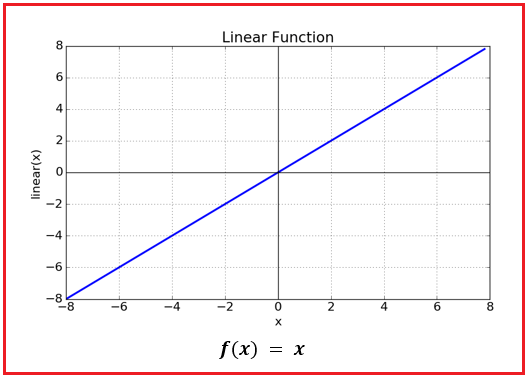Range – (- infinity to infinity)

###### 2. Binary Step Function –

One of the most basic types of activation functions is the step function. In this, a threshold value is taken into consideration, and the neuron is triggered if the value of the net input, let’s say y, exceeds the threshold. Mathematically expressed as,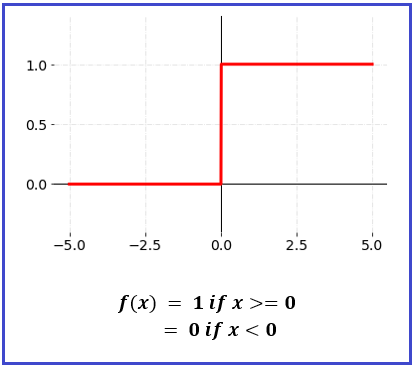##### Non-Linear Activation Function in Artificial Neural Network –

The most often utilized activation functions are nonlinear activation functions. The activation function transforms the input in a non-linear way, enabling it to learn and carry out more difficult tasks.

It facilitates the model’s generalization or adaptation to a variety of data and facilitates output differentiation. An activation that is nonlinear Functions are typically categorized according to their range or curvature-

##### 1. Sigmoid Activation Function –

The Sigmoid Function curve has an S-shaped appearance. We employ the sigmoid function primarily because it occurs between (0 to 1). As a result, it is particularly used for models whose output is a probability prediction. The sigmoid is the best option because anything has a probability that only occurs between 0 and 1.

The function might take numerous forms and is differentiable. Therefore, we can determine the sigmoid curve’s slope between any two points.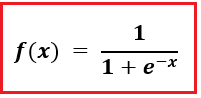Range: 0 to 1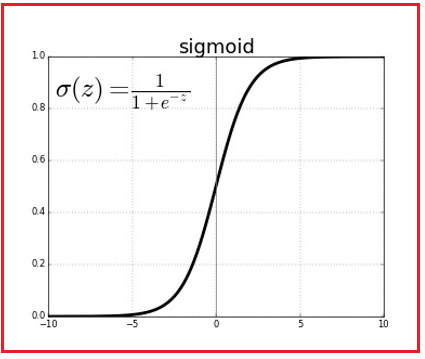##### 2. Tanh Activation Function –

Tanh is like a better version of a logistic sigmoid. The tanh function’s range is from (-1 to 1). Tanh is sigmoidal as well (s-shaped). The positive aspect of this is that the zero inputs will be mapped near zero and the negative inputs will be highly negative in the tanh graph. The function might take numerous forms. The tanh function is mostly used to classify data into two groups.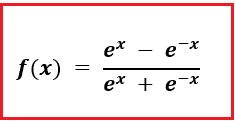Range: -1 to 1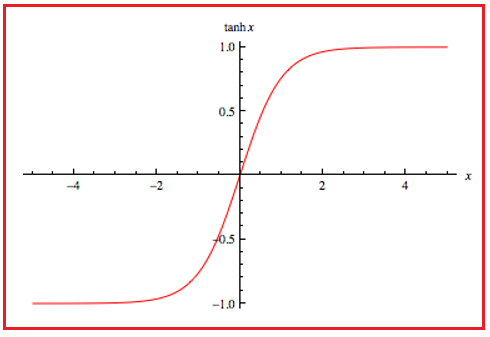##### 3. ReLU (Rectified Linear Unit) Activation Function –

Currently, the ReLU is the activation function that is employed the most globally. Since practically all convolutional neural networks and deep learning systems employ it. As seen, the ReLU is only partially fixed (from the bottom). When x is less than zero, f(x) equals zero, and when x is more than or equal to zero, f(x) equals x.

However, the problem is that all the negative values instantly become zero, which reduces the model’s capacity to effectively fit or train from the data. This means that any negative input to the ReLU activation function immediately becomes zero in the graph, which has an impact on the final graph by improperly mapping the negative values.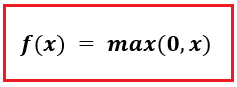Range: 0 to infinity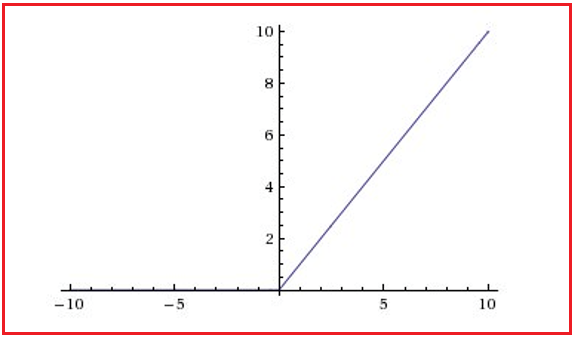##### 4. Leaky ReLU Activation Function –

A better variant of the ReLU function is what the leaky ReLU function is. We define the Relu function as a small linear component of x rather than as 0 for x less than 0. It is mathematically expressed as: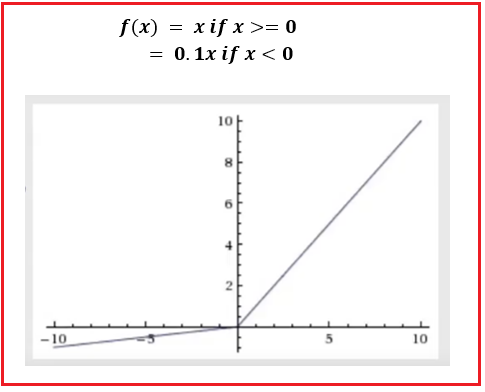In the next article, I am going to discuss How Artificial Neural Network Work. Here, in this article, I try to explain the Activation Functions in Artificial Neural Networks. I hope you enjoy this Activation Functions in Artificial Neural Network article. Please post your feedback, suggestions, and questions about Activation Functions in the Artificial Neural Network article.

### 1 thought on “Activation Functions in Artificial Neural Network”

1.Guys,
Please give your valuable feedback. And also, give your suggestions about this Activation Functions in Artificial Neural Networks concept. If you have any key points related to the Activation Functions in Artificial Neural Networks, you can also share the same.